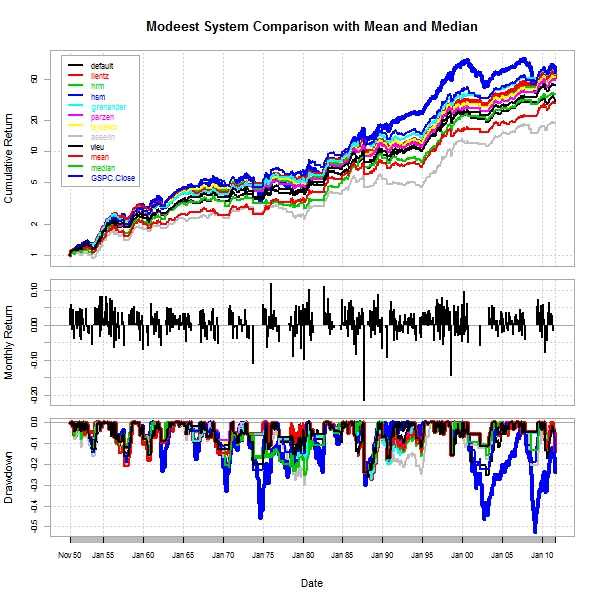Want to share your content on R-bloggers? click here if you have a blog, or here if you don't.

I have no idea who originated the idea of using moving averages to determine entry and exit points in a trading system.  I do know that Mebane Faber (briefly discussed in Shorting Mebane Faber) has recently popularized the notion through his >75,000 download SSRN working paper

Faber, Mebane T., A Quantitative Approach to Tactical Asset Allocation (February 17, 2009). Journal of Wealth Management, Spring 2007. Available at SSRN: http://ssrn.com/abstract=962461

and his book

The general measures of central tendency are mean (average), median, and mode.  When I spotted the modeest package in my Planet R feed and then read When Are Averages Useless at ExploringDataBlog, I thought I should at least try all these mode options as a potential replacement to the Faber 10-month moving average.  I must confess that I do not understand all of these various mode options, but through the beauty of R I really don’t need to completely understand them to apply them.

AS ALWAYS THIS IS NOT INVESTMENT ADVICE.  THIS IS MERELY AN ILLUSTRATION AND MOST LIKELY WILL RESULT IN SIGNIFICANT LOSSES.

After some very basic tests, it appears hsm works most consistently best on equity indexes and even works on Crazy RUT.  These modes might offer an attractive alternative to the far more popular moving average.From TimelyPortfolioFrom TimelyPortfolio

`require(modeest)require(quantmod)require(PerformanceAnalytics)   getSymbols("^GSPC",from="1896-01-01",to=Sys.Date())GSPC.monthly <- to.monthly(GSPC)[,4]index(GSPC.monthly) <- as.Date(index(GSPC.monthly))   #width will represent number of months for modewidth = 10for (i in width:NROW(GSPC.monthly)) {	#not real proud of this code	#please feel free to comment with improvements	m.default <- mlv(GSPC.monthly[(i-width):i])	m.lientz <- mlv(GSPC.monthly[(i-width):i], method = "lientz", bw=0.01)	m.hrm <- mlv(GSPC.monthly[(i-width):i], method = "hrm", bw=0.01)	m.hsm <- mlv(GSPC.monthly[(i-width):i], method = "hsm",tie.action = "mean")	m.grenander <- mlv(GSPC.monthly[(i-width):i], method = "grenander", p = 0.5)	m.parzen <- mlv(GSPC.monthly[(i-width):i], method = "parzen", kernel = "gaussian")	m.tsybakov <- mlv(GSPC.monthly[(i-width):i], method = "tsybakov")	m.asselin <- mlv(GSPC.monthly[(i-width):i], method = "asselin")	m.vieu <- mlv(GSPC.monthly[(i-width):i], method = "vieu")   m.all <- c(as.numeric(m.default), as.numeric(m.lientz),		as.numeric(m.hrm), as.numeric(m.hsm), as.numeric(m.grenander),		as.numeric(m.parzen), as.numeric(m.tsybakov),		as.numeric(m.asselin), as.numeric(m.vieu))	ifelse(i==width, m.hist <- m.all, m.hist <- rbind(m.hist,m.all))}   m.xts <- as.xts(as.data.frame(m.hist),order.by=index(GSPC.monthly[(width):NROW(GSPC.monthly),]))m.xts <- merge(m.xts,runMean(GSPC.monthly,n=10),runMedian(GSPC.monthly,n=10))colnames(m.xts) <- c("default","lientz","hrm","hsm","grenander","parzen",	"tsybakov","asselin","vieu","mean","median")GSPC.mode <- merge(GSPC.monthly,m.xts)   #there has to be a much cleaner way to accomplish thissignal <- GSPC.mode[,2:NCOL(GSPC.mode)]ret <- GSPC.mode[,2:NCOL(GSPC.mode)]for (i in 1:NCOL(signal)) {	signal[,i] <- ifelse(GSPC.mode[,1] > GSPC.mode[,i+1],1,0)	ret[,i] <- lag(signal[,i],k=1) * ROC(GSPC.mode[,1],type="discrete",n=1)}ret <- merge(ret,	ROC(GSPC.mode[,1],type="discrete",n=1))   #jpeg(filename="modeest performance summary.jpg",#	quality=100,width=6.25, height = 6.25,  units="in",res=96)charts.PerformanceSummary(ret,ylog=TRUE,lwd=c(rep(2,9),rep(4,3)),	main="Modeest System Comparison with Mean and Median")#dev.off()   t(table.AnnualizedReturns(ret))   #jpeg(filename="modeest risk return.jpg",#	quality=100,width=6.25, height = 6.25,  units="in",res=96)chart.RiskReturnScatter(ret)#dev.off()`